Share

# Concept of Work

### Work:

“The work done by the force is defined to be the product of component of the force in the direction of the displacement and the magnitude of this displacement.”

The work W done by a constant force F when its point of application undergoes a displacement s is defined to be
W=bar F.bar s=F s cos θ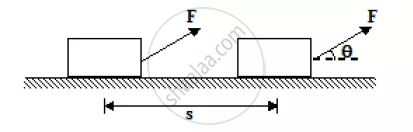where θ is the angle between bar F and bar s as indicated in figure. Only the component of bar F along s, that is fcos θ, contributes to the work done.
Work is a scalar quantity and its SI unit is the joule (J). From the above equation,  we see that
1 J = 1 Nm = 107 erg
In terms of rectangular components, the two vectors are
bar F= F_x hat i + F_y hat j + F_ z hat k
and bar s = Δx hat i +Δ y hat j+Δ z hat k
hence, the above equation may be written as
W=F_x Δx + F_y Δy + F_z Δz

The work done by a given force on a body depends only on the force, the displacement, and the angle between them. It does not depend on the velocity or the acceleration of the body, or on the presence of other forces.

When several forces act on a body one may calculate the work done by each force individually. The net work done on the body is the algebraic sum of individual contributions.
W_"net" = bar F_1. bar S_1 + bar F_2. bar S_2 +.........+ bar F_n. bar S_n or
W_"net" = W_1 + W_2 +............ + W_n

No work is done if:

1. The displacement is zero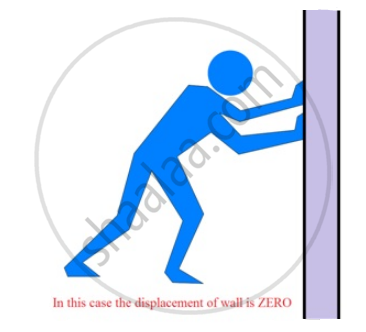2. The force is zero.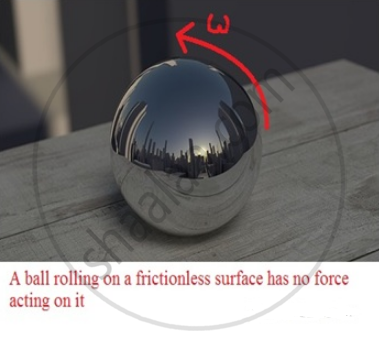3. The force and displacement are mutually perpendicular.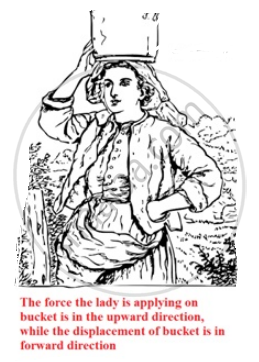Positive, Negative and Zero Work:-
Work done by a force may be positive or negative depending on the angle θ between the force and displacement. If the angle θ is acute (θ < 90^o), then the work done is positive and the component of force is parallel to the displacement.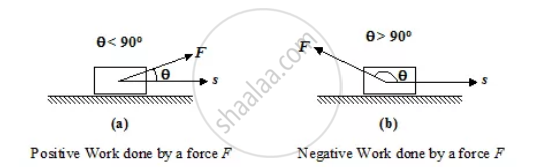If the angle θ is obtuse (θ > 90^o), the component of force is antiparallel to the displacement and the work done by force is negative.

Application 1
When a person lifts a body from the ground to some higher position, the work done by the lifting force (i.e. the force applied by the person) is positive since force (vector) and displacement (vector) are along the same (vertically upward) direction and hence,
θ = 0 cos θ =1
However, the work done by the gravity (or the force by the earth on the body) is negative since force (vector) and displacement (vector) are oppositely directed and hence
θ = 180o cos θ = −1.

Application 2
A box is moved over a horizontal path by applying force F = 60 N at an angle θ = 30o to the horizontal. What is the work done during the displacement of the box over a distance of 0.5 km.
Solution:
By definition, W = F s cos θ
Here F = 60 N; s = 0.5 km = 500m; θ= 30o
W = (60)(500) cos30o = 26 kJ

S